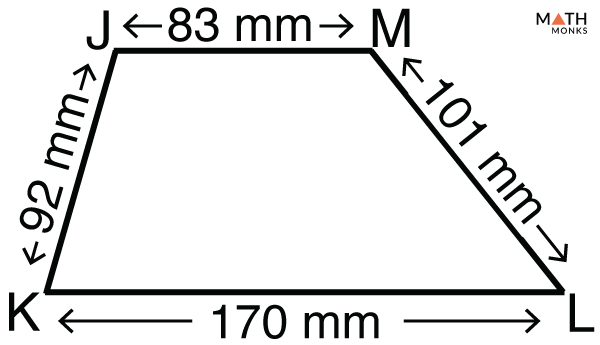# Perimeter of a Trapezoid

The perimeter of a trapezoid is the total distance covered around its edge. The trapezoid, a member of the quadrilateral family, is generally an irregular polygon. So we sum up all its four sides to calculate its perimeter. The perimeter is always linear. So its units are also linear, such as cm, in, m, ft, or yd.

## Formula

The basic formula to how to find the perimeter of a trapezoid is given below:

Let us solve an example to understand the concept better.What is the perimeter of a trapezoid JKLM?

Solution:

As we know,
Perimeter (P) = a + b + c + d, here a = 170 mm, b = 83 mm, c = 92 mm, d = 101 mm
∴ P = 170 + 83 + 92 + 101
= 446 mmWhat is the perimeter of an isosceles trapezoid whose bases are 34.4 cm and 16.4 cm, and legs are 12.5 cm each?

Solution:

As we know,
Perimeter (P) = a + b + 2c, here a = 34.4 cm, b = 16.4 cm, c = 12.5 cm
∴ P = 34.4 + 16.4 + 2 × 12.5
= 75.8 cm